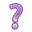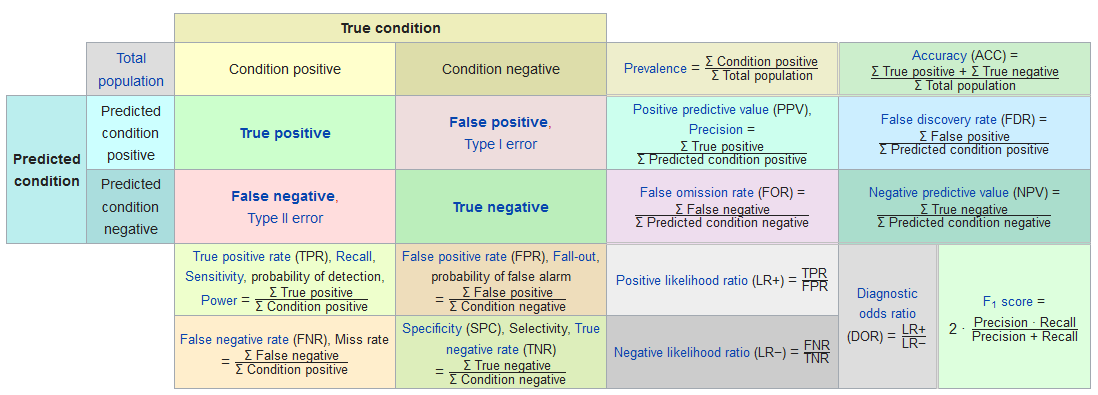## 11.2 Confusion Matrix

A confusion Matrix is the 2x2 table that compares the predicted classes to the true classes.

table(plot.mpp$pred.class, plot.mpp$truth)
##
##                 Not Depressed Depressed
##   Not Depressed           195        35
##   Depressed                49        15

This table was generated by drawing a random Bernoulli variable with probability $$p_{i}$$. This assumes that probabilities can range from [0,1], but if you look at the plots above, the predicted probabilities max out around 0.5.

Often we adjust the cutoff value to improve accuracy. This is where we have to put our gut feeling of what probability constitutes “high risk”. For some models, this could be as low as 30%. It’s whatever the probability is that optimally separates the classes. This is an important tuning parameter because since the models we build are only based on data we measured, often there are other unmeasured confounding factors that affect the predicted probability. So our predictions don’t span the full range from [0,1].Using the above plots, where should we put the cutoff value? At what probability should we classify a record as “depressed”?

There are many different types of criteria that can be used to find the optimal cutoff value. But first we need to understand the expanded borders of a [Confusion Matrix].Confusion matrix. Credit: Wikipedia

Using the confusionMatrix function inside the caret package performs all these calculations for us.

You must specify what the ‘event’ is. This is also another place where the factor ordering of binary variables can cause headache. Another reason to control your factors!
caret::confusionMatrix(plot.mpp$pred.class, plot.mpp$truth, positive="Depressed")
## Confusion Matrix and Statistics
##
##                Reference
## Prediction      Not Depressed Depressed
##   Not Depressed           195        35
##   Depressed                49        15
##
##                Accuracy : 0.7143
##                  95% CI : (0.659, 0.7652)
##     No Information Rate : 0.8299
##     P-Value [Acc > NIR] : 1.0000
##
##                   Kappa : 0.0892
##
##  Mcnemar's Test P-Value : 0.1561
##
##             Sensitivity : 0.30000
##             Specificity : 0.79918
##          Pos Pred Value : 0.23438
##          Neg Pred Value : 0.84783
##              Prevalence : 0.17007
##          Detection Rate : 0.05102
##    Detection Prevalence : 0.21769
##       Balanced Accuracy : 0.54959
##
##        'Positive' Class : Depressed
## 
• 195 people were correctly predicted to not be depressed (True Negative)
• 49 people were incorrectly predicted to be depressed (False Positive)
• 10 people were incorrectly predicted to not be depressed (False Negative)
• 15 people were correctly predicted to be depressed (True Positive)